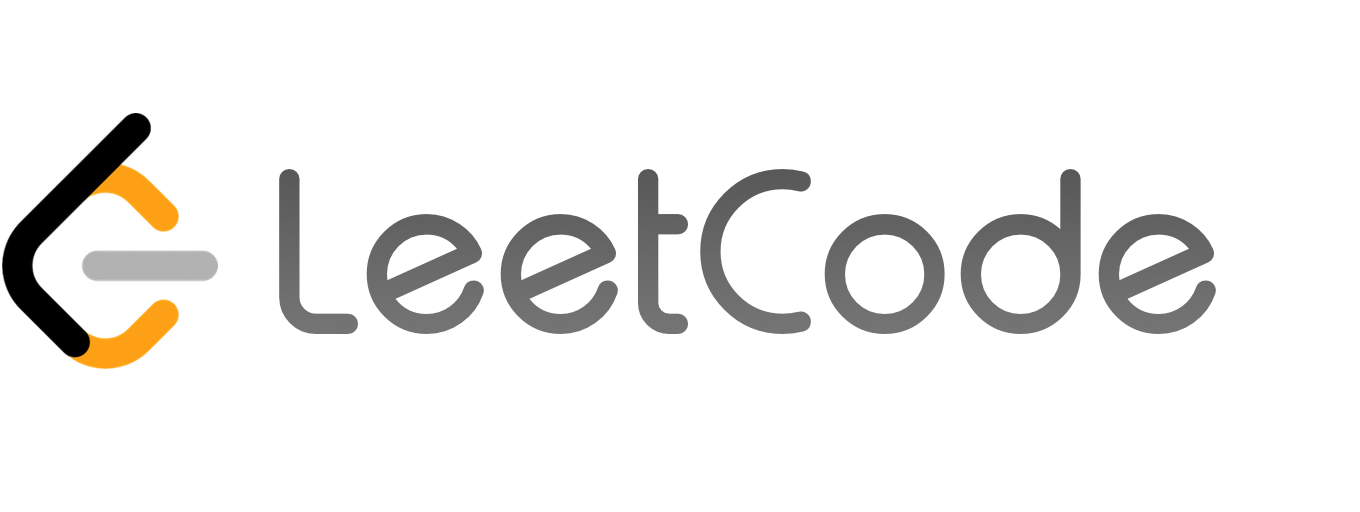LeetCode面试系列 第3天：No.67 - 二进制数求和_极客玩家大白

# LeetCode面试系列 第3天：No.67 - 二进制数求和

Bravo Yeung 2019/09/14 00:00:00

Leetcode面试题### 题目描述

``````1
2

``````

``````1
2

``````

``````1
2
3
4
5
6
class Solution:
def addBinary(self, a: str, b: str) -> str:
num1 = int(a, 2)
num2 = int(b, 2)
sum0 = num1 + num2
return format(sum0, 'b')
``````

ps: 这里和的变量名使用`sum0`是因为 `sum` 是 Python 中内置的关键字。``````1
2
3
4
5
6
7
8
9
10
class Solution:
def addBinary(self, a: str, b: str) -> str:
num1 = int(a, 2)
num2 = int(b, 2)
sum0 = num1 + num2
return format(sum0, 'b')

# 测试
sol = Solution()